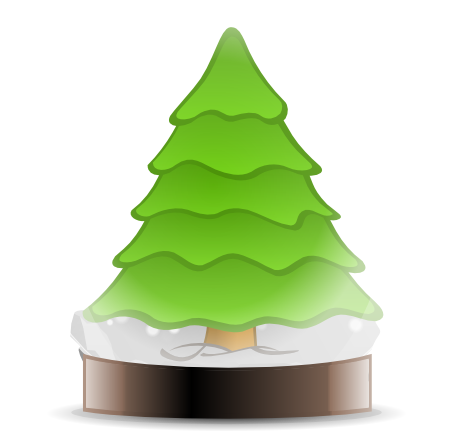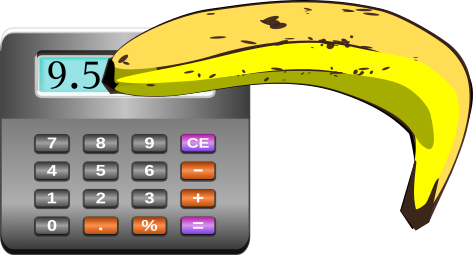# Exam-Style Questions.

## Problems adapted from questions set for previous Mathematics exams.

### 1.

GCSE Higher

A number, $$n$$, when rounded to two decimal places is 7.32

Express the error interval for $$n$$ using inequalities.

### 2.

GCSE Higher

A number, $$n$$, is rounded to 2 decimal places.

The result is 13.77

Complete the error interval for $$n$$.

### 3.

GCSE Higher

The height of a tree is 9 metres to the nearest metre.(a) Complete the error interval for the height of the tree.

$$........ m \le height \lt ........ m$$

The height of a different tree is 7 metres to the nearest metre.

Mr Schneebly says "The sum of the heights of the two trees is 17 metres to the nearest metre."

(b) Give an example to show that he could be correct.

### 4.

GCSE Higher

(a) Work out an estimate for the value of $$\sqrt{48.3 \times 82.01}$$.

$$(3.4)^5 = 454$$ correct to 3 significant figures.

(b) Find the value of $$(0.034)^5$$ correct to 3 significant figures.

(c) Find the exact value of $$3^{–3}$$

### 5.

GCSE Higher

Ralph used his calculator to work out the value of C, the circumference of a circle. Unfortunately he put his banana down on his desk so that it covered most of the calculator screen. All that can be seen are the first two digits of the answer.Complete the error interval for C

$$. . . . . . . . . . \le C \lt . . . . . . . . . .$$

### 6.

GCSE Higher

(a) Calculate the upper bound for the value of A giving your answer correct to 6 significant figures if:

$$A = \frac{3b}{2c^2}$$

$$b = 7.4$$ correct to 2 significant figures and $$c = 4.38$$ correct to 3 significant figures.

(b)The lower bound for the value of A is 0.573376 correct to 6 significant figures.

By considering bounds, write down the value of A to a suitable degree of accuracy. You must give a reason for your answer.

If you would like space on the right of the question to write out the solution try this Thinning Feature. It will collapse the text into the left half of your screen but large diagrams will remain unchanged.

The exam-style questions appearing on this site are based on those set in previous examinations (or sample assessment papers for future examinations) by the major examination boards. The wording, diagrams and figures used in these questions have been changed from the originals so that students can have fresh, relevant problem solving practice even if they have previously worked through the related exam paper.

The solutions to the questions on this website are only available to those who have a Transum Subscription.

Exam-Style Questions Main Page

Search for exam-style questions containing a particular word or phrase:

To search the entire Transum website use the search box in the grey area below.